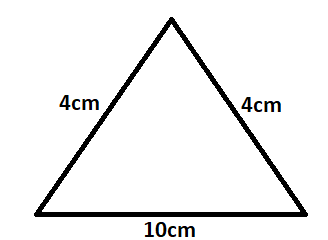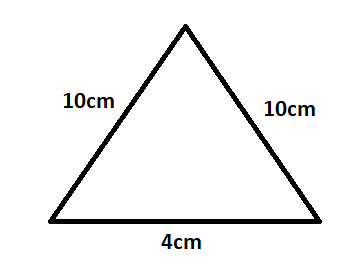Courses
Courses for Kids
Free study material
Offline Centres
MoreLast updated date: 24th Nov 2023
Total views: 280.2k
Views today: 3.80k

# If two sides of an isosceles triangle are 4cm and 10cm. then the length of the third side isA) 4cmB) 10cmC) 4cm or 10cmD) None of theseVerified
280.2k+ views
Hint: Given is an isosceles triangle. We know that the isosceles triangle has two sides that are the same. Thus two sides given are 4cm and 10cm. so the third side should be of measurement that is either of the two sides given.

Complete step by step solution:
Given is an isosceles triangle.
We know that this type of triangle has two sides of the same measurement.
Thus given the sides are $4cm$ and $10cm$.
Thus if the third side is $4cm$ then that triangle will be an isosceles triangle.Also if the third side is 10cm then also it is an isosceles triangle.Thus, option (C) is the correct option.

Note:
Note that the only restriction for a triangle to be an isosceles is any two sides should be of same length or we can say same dimensions.
Like for a triangle to be the right angle, one of the angles is of ${90^ \circ }$.
For a scalene triangle, all the sides are different.
For an equilateral triangle all the sides are the same and all the angles are of ${60^ \circ }$.